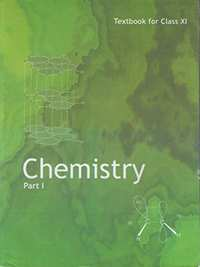Share

# NCERT solutions for Class 11 Chemistry Textbook chapter 1 - Some Basic Concepts of Chemistry [Latest edition]

Textbook page

#### Chapters## Chapter 1: Some Basic Concepts of Chemistry

### NCERT solutions for Class 11 Chemistry Textbook Chapter 1 Some Basic Concepts of Chemistry [Page 22]

Q 1.2 | Page 22

Calculate the mass percent of different elements present in sodium sulphate (Na2SO4).

## Chapter 1: Some Basic Concepts of Chemistry## NCERT solutions for Class 11 Chemistry Textbook chapter 1 - Some Basic Concepts of Chemistry

NCERT solutions for Class 11 Chemistry Textbook chapter 1 (Some Basic Concepts of Chemistry) include all questions with solution and detail explanation. This will clear students doubts about any question and improve application skills while preparing for board exams. The detailed, step-by-step solutions will help you understand the concepts better and clear your confusions, if any. Shaalaa.com has the CBSE Class 11 Chemistry Textbook solutions in a manner that help students grasp basic concepts better and faster.

Further, we at Shaalaa.com provide such solutions so that students can prepare for written exams. NCERT textbook solutions can be a core help for self-study and acts as a perfect self-help guidance for students.

Concepts covered in Class 11 Chemistry Textbook chapter 1 Some Basic Concepts of Chemistry are Importance of Chemistry, Historical Approach to Particulate Nature of Matter, Nature of Matter, The International System of Units (Si), Mass and Weight, Scientific Notation, Significant Figures, Dimensional Analysis, Law of Conservation of Mass, Law of Definite Proportions, Law of Multiple Proportions, Gay Lussac’S Law of Gaseous Volumes, Avogadro Law, Dalton'S Atomic Theory, Atomic Mass, Average Atomic Mass, Molecular Mass, Formula Mass, Mole Concept and Molar Masses, Percentage Composition, Stoichiometry and Stoichiometric Calculations - Introduction, Stoichiometry and Stoichiometric Calculations - Limiting Reagent, Stoichiometry and Stoichiometric Calculations - Reactions in Solutions, Some Basic Concepts of Chemistry Numericals.

Using NCERT Class 11 solutions Some Basic Concepts of Chemistry exercise by students are an easy way to prepare for the exams, as they involve solutions arranged chapter-wise also page wise. The questions involved in NCERT Solutions are important questions that can be asked in the final exam. Maximum students of CBSE Class 11 prefer NCERT Textbook Solutions to score more in exam.

Get the free view of chapter 1 Some Basic Concepts of Chemistry Class 11 extra questions for Class 11 Chemistry Textbook and can use Shaalaa.com to keep it handy for your exam preparation